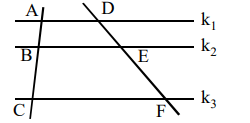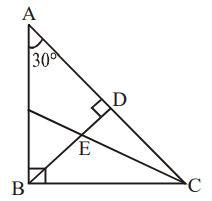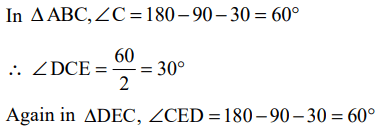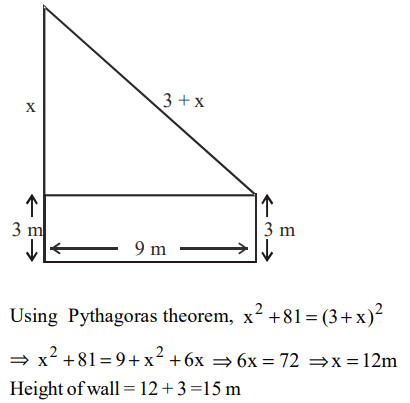## Geometry Questions and Answers Part-1

1. $k_{1},k_{2},k_{3}$   are parallel lines. AD = 2 cm, BE = 8 cm and CF = 32 cmThen
I. (AB) × (EF)
II. (BC) × (DE)
a) I > II
b) I < II
c) I = II
d) Nothing can be said

Explanation:2. Which one of the following cannot be the ratio of angles in a right angled triangle?
a) 1 : 2 : 3
b) 1 : 1 : 2
c) 1 : 3 : 6
d) None of these

Explanation: Clearly option (a) shows the angles would be 30, 60 and 90. It can be the ratio of angle in a right angled triangle.
Option (b) shows the angles would be 45, 45 and 90, then it can be the ratio of angle in a right angled triangle.
But option (c) cannot form the ratio of angles of right angled triangle.

3. In $\triangle ABC ,AB \bot BC$    and $BD \bot AC$   . And CE bisects the angle C. $\angle A=30^{\circ}$ . What is $\angle CED$a) 30°
b) 60°
c) 45°
d) 65°

Explanation:4. In the given figure, AB is diameter of the circle and the points C and D are on the circumference such that $\angle CAD=30^{0}$   and $\angle CBA=70º$    . What is the measure of $\angle ACD$a) 40°
b) 50°
c) 30°
d) 90°

Explanation:5. The length of a ladder is exactly equal to the height of the wall it is resting against. If lower end of the ladder is kept on a stool of height 3 m and the stool is kept 9 m away from the wall the upper end of the ladder coincides with the tip of the wall. Then, the height of the wall is
a) 12m
b) 15m
c) 18m
d) 11m

Explanation:6. In triangle ABC, angle B is a right angle. If (AC) is 6 cm, and D is the mid - point of side AC. The length of BD isa) 4 cm
b) $\sqrt{6}cm$
c) 3 cm
d) 3.5 cm

Explanation:7. The points of intersection of three lines , 2X + 3Y - 5 = 0, 5X - 7Y + 2 = 0, 9X - 5Y - 4 = 0,
a) form a triangle
b) are on lines perpendicular to each other
c) are on lines parallel to each other
d) are coincident

Explanation:8. If ABCD is a square and BCE is an equilateral triangle, what is the measure of the angle DEC?a) 15°
b) 30°
c) 20°
d) 55°

Explanation:9.AB is the diameter of the given circle, while points C and D lie on the circumference as shown. If AB is 15 cm, AC is 12 cm and BD is 9 cm, find the area of the quadrilateral ACBDa) 54 $cm^{2}$
b) 216 $cm^{2}$
c) 162 $cm^{2}$
d) None of these

Explanation:10. In the given figure, EADF is a rectangle and ABC is a triangle whose vertices lie on the sides of EADF.
AE = 22, BE = 6, CF = 16 and BF = 2
Find the length of the line joining the mid-points of the sides AB and BC.a) $4\sqrt{2}$
b) 5
c) 3.5
d) None of these